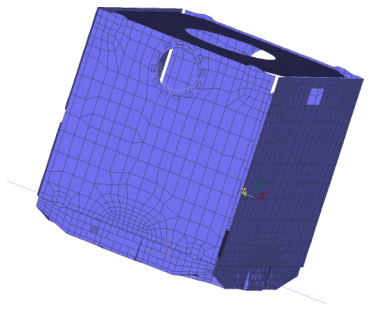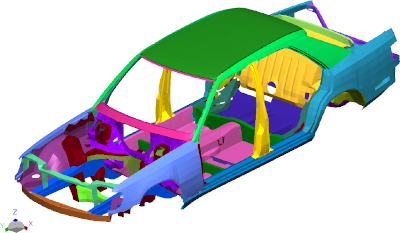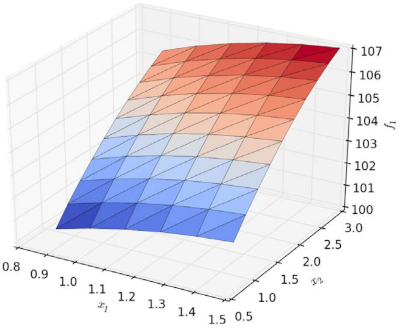# Robust Optimum Design

In order to achieve a robust design, it is not sufficient to perform a deterministic optimization:

• Optimization often leads to reduced safety margins.
• The optimized design may have other critical parameters than the inital design.
• A 'reliable' optimum may be different than a deterministic one.

The proposed solution is a combination of optimization and reliability analysis. For this combination two different approaches are available:

Two step approach
• Basic design
• FE-Analysis (e.g. static analysis)
• Reliability analysis
• Optimization taking into account reliability
• Optimization
• Reliability analysis of optimized design
• If not sufficient:
• Modify the design model according to reliability results
• Repeat the optimization and the reliability loop
One step approach
• Combined optimization and reliability analysis
• Reliability as design constraint in optimization

For the one-step approach, the interplay of design variables in optimization and basic variables in reliability analysis is as follows:

• Design Variables
• define the design state of the structure
• may be modified by the optimizer
• may be assigned to one of the following types:
• Deterministic design variable
• Deterministic mean value of a stochastic design variable
• Basic Variables
• Basic variables define the stochastic properties of the problem
• Following types of basic variables are possible:
• Stochastic properties of the structure
• Stochastic design variable with deterministic mean value
• Limit state function parameter
• Parameter of another basic variable

In the one step approach, there two different states belong to each design point:

• Design state
• Limit state

For each state one FE-analysis is necessary. Therefore, for each step during optimization at least two FE-analyses must be performed.

The design state is the actual optimization state. It is given by:

• Actual values of design variables
• Mean values of basic variables

The objective function is evaluated for the design state. The design constraints are evaluated for the design state. The final design state must fulfil the design constraints.

The limit state describes for a given design state the corresponding failure state. It is given by:

• Actual values of design variables
• Actual values of basic variables

The limit state function is evaluated for the limit state. The design constraints are meaningless for the limit state, e.g. the limit values for the limit state function and the limit values for the design constraints are different.

The combined analysis gives the following results:

• Final design state
• Objective function value
• Design variable values
• Elasticities of design variables with respect to objective function
• Probability of failure
• Values of active constraints
• Final limit state
• Basic variable values
• Parameter sensitivities of the limit state function
• Always available:
• Selected data for each iterationPROTEUS satellite (Alcatel Space S.A.) with 28 design variables and 30 stochastic basic variables.

BasicOptimizedOne Step
Mass:M324.8308.9312.5
Maximum stress:σ9.6 · 1071.2 · 1085.5 · 106
Failure probability:Pf8.2 · 10-74.2 · 10-51.0 · 10-6
Failure rate (one of):1.2 Mio238091 Mio.Influence of thickness variation of roof/floor on 1 mode